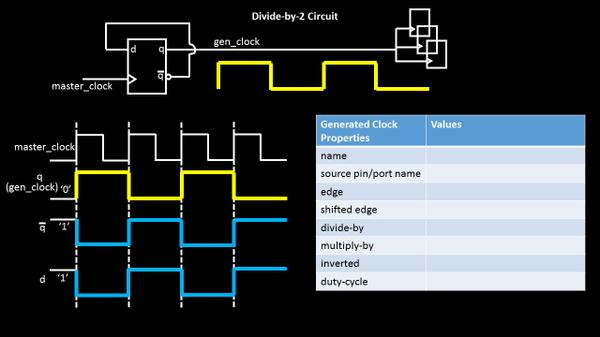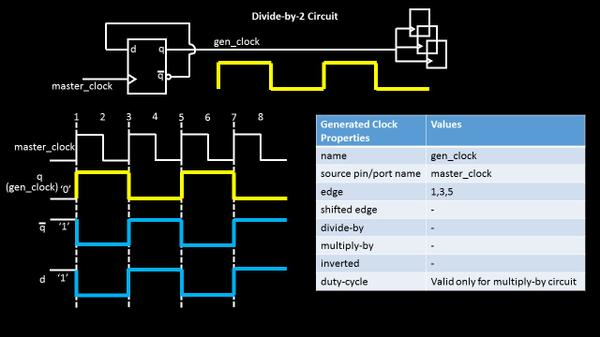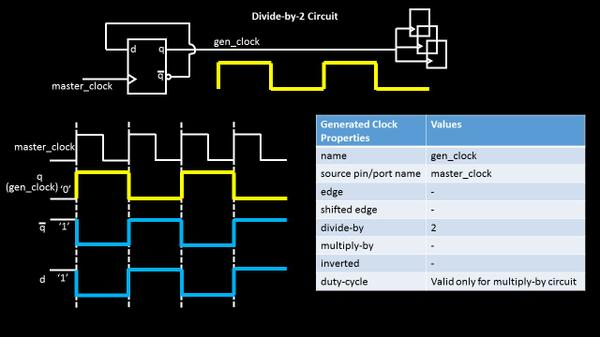# Generated clock & master clock.. Let’s make it simple!! – Part 1

Hello

I get this one occasionally … not particularly about the concept, but about the ways we can create a generated clock definition. Too many options make it too complex… But you know what, with a handful of images, this topic becomes way too simple… Let me show how? Start with below simple divide-by-2 circuit and try to define it in all ways possible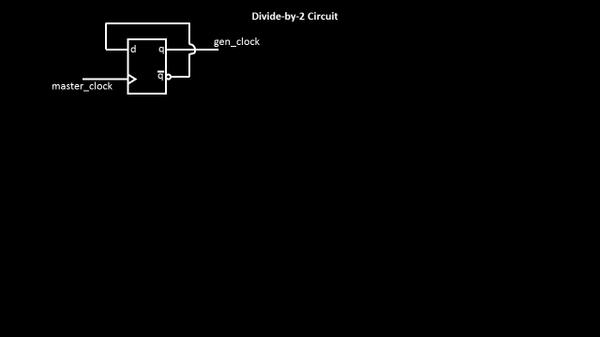I believe, best way to understand any topic is the ‘graphical way’. So, let me show you how the input and output wave-forms look like, in below image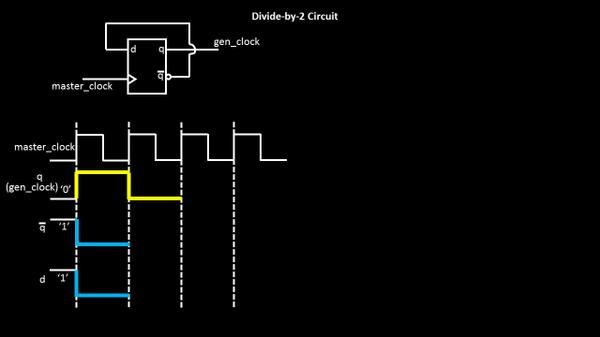If you start with master clock of say 1ns period, the output is expected of 2ns period (frequency divide-by-2). So at the first rising edge of clock (assuming the initial state of circuit is ‘q’ = 0 and ‘d’ = 1), the data at ‘d’ pin will be propagated at ‘q’ output (since it’s a rise-edge triggered flop),  and ‘q’ becomes ‘1’, ‘q_bar’ becomes ‘0’, and ‘d’ is at ‘0’ (‘q_bar’ is connected to ‘d’). This is shown in above image. While, we keep doing this, the state of the circuit changes at every rise edge of master_clock, and below is what you get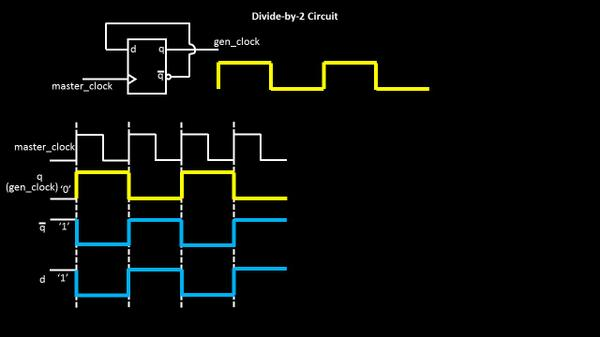Now that was the digital hardware part of it. Let me tell you a secret of ‘static timing analysis’ of ASIC…We are ‘static’. We need definitions. We understand software language. And we will not change J . You are right in thinking.. We are adamant on this J
Designer – Ok, so let me speak to you in your language. Let me write a define this new ‘gen_clock’ for you so you understand it and use it
STA – you better do !!